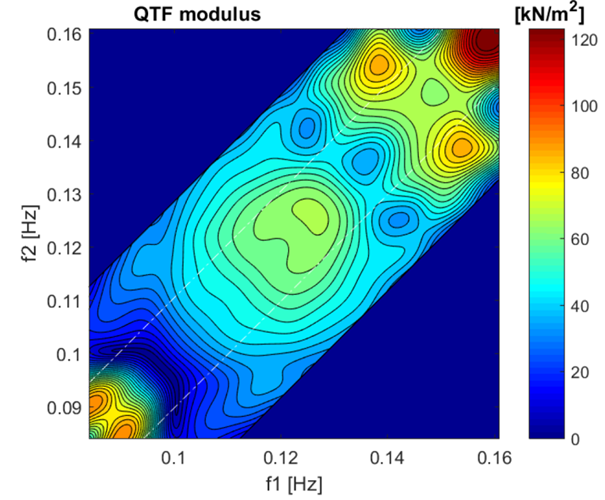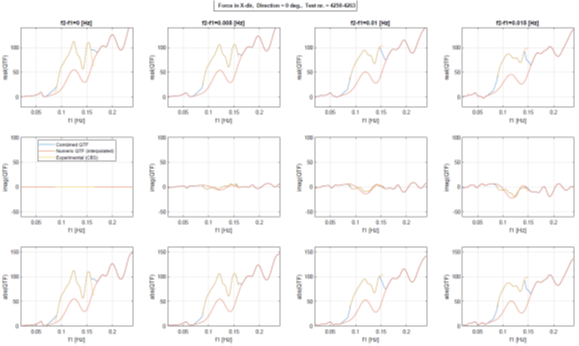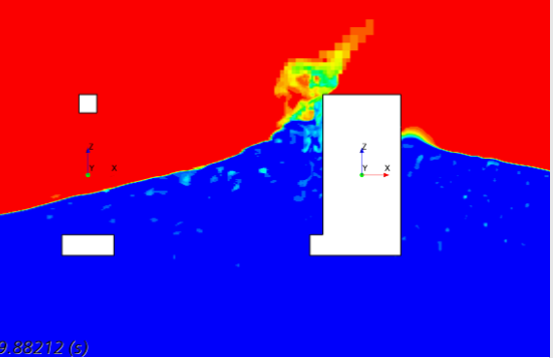# WP2: Low frequency hydrodynamic loadsFigure 1: Absolute value of the surge wave drift QTF (Hs = 6.2 m, Tp = 9.0 s)

This WP focus on methods to calculate low frequency (LF) motions of floating wind turbines (FWTs), including conditions with combined waves and current and moderate and severe seastates. The problem involves prediction of (nonlinear) LF wave exciting forces and prediction of LF damping.

Fully rational methods accounting for all relevant nonlinear effects are not available today. Therefore, the objective is to investigate different approximations, conclude on the validity and, as a result, recommend calculation procedures for FWTs. The WP is organized in four tasks as described below.

Task 2.1: Post analysis of experimental data

Post-processing of the model test data to be acquired in WP4 with a second order identification method known as cross bi spectral analysis. This procedure identifies empirical full quadratic transfer functions of the wave exciting forces. It allows for identification of wave-current effects on full QTFs (analysis of tests with and without current), as well for identification of higher than second order effects (analysis of tests with increasing wave heights). Figure 1 presents one example of an empirical surge QTF identified from a model test.Figure 1: Absolute value of the surge wave drift QTF (Hs = 6.2 m, Tp = 9.0 s).

Task 2.2: Nonlinear potential flow theory

Application of different approximation methods to calculate nonlinear wave drift forces and LF damping, and assess the results by comparison with model test data. Some of the approximations are implemented and tested within the project. Figure 2 compares several diagonals of the surge wave force QTF calculated by a second order potential flow code with coefficients identified from model tests. The plots illustrates the underestimation by a state of the art code due to neglecting wave-current effects and higher than 2nd order effects.Figure 2: Surge wave drift QTF diagonals (Hs = 6.2 m, Tp = 9.0 s). Identification of limitations from 2nd order predictions by comparing with empirical coefficients.